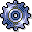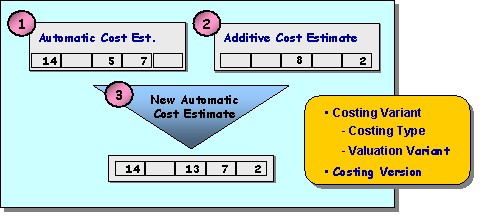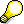Additive CostsUse

You use additive costing to add costs manually to a material cost estimate when they cannot be calculated by the system. Examples of such costs are freight charges, insurance costs, stock transfer costs, incomplete or changed BOMs, and routings.

As a rule, costing calculates the costs of a material on the basis of the quantity structure. This type of cost estimate is performed automatically by the system. However, you can also manually enter estimated values for costs that cannot be calculated by the system. This allows you to add costs to a cost estimate that was calculated automatically.

Prerequisites

The main prerequisite for including additive costs in an automatic cost estimate is that you carry out both automatic and additive costing on the basis of the same costing variant and costing version.

In addition, you need to carry out or check the following settings in Customizing:

• To ensure that the additive costs are included, set the indicator Incl. additive costs for each valuation strategy in the valuation variant in Customizing for Product Cost Planning.
• Specify the following through the costing variant in Customizing for Product Cost Planning:
• Whether any existing additive costs are to be included in cost estimates that you create with this costing variant
• Whether the additive costs are to be included when calculating overhead
• Whether the additive costs are to be included when transferring stock to another plant
• You create separate cost components in Customizing for Product Cost Planning for those costs which you enter with the additive cost estimate, for example if you want to include the following costs in the cost estimate:
• Special direct costs of production
• Costs for materials for which no BOM has yet been created
• Costs for work sequences for which no routing has yet been created

For more information about creating costing variants and valuation variants, and also updating additive costs in cost components, see the Implementation Guide (IMG) for Product Cost Controlling.

Features

The following graphic shows the link between the automatic cost estimate and the additive cost estimate.When you cost materials, the system determines the BOM for the material, and selects a price for the valuation of the material components through the valuation variant. If you set the Incl. additive costs indicator in the valuation variant, the system looks for any existing additive cost estimates for the material. The system adds the costs entered manually to the costs calculated by the system. The costs in the automatic cost estimate and the additive cost estimate are added together for each cost component.

If you enter or change costs manually for a material, these costs are not automatically rolled up in the BOM structure. You must first create a new material cost estimate or execute a new costing run so that the changes are included and rolled up. If additive costs have been included, the system sets the Additive costing exists indicator in the overview screen of the cost estimate. You can then update the costing results in the material master .The additive costs are added in their line item form to the itemization of a cost estimate with quantity structure; in doing so item categories S (total) and T (text) are not transferred. If the costing item has a cost component split, then the cost element under which the item is entered is transferred, not the cost component split itself.

Additive cost estimates with dates that are not within the period of validity of the automatic cost estimate are not included in the automatic cost estimate.

The valuation date specifies the date for which the prices are selected. If a price is not valid on the valuation date, it cannot be selected. For more information, see Date Control.You enter the additive costs manually in the form of a unit cost estimate. When you create additive costs using unit costing, you can use the items of an existing additive cost estimate as a reference.